Connect with us

# Measuring Return on Investment [5 Scenarios]Published

on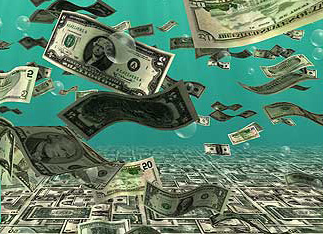Measuring investment performance can be as simple as reading a comic strip or as perplexing as reading a book on nuclear physics. The primary measure of investment performance is the annual rate of return on investment, or ROI. Return in ROI refers to: the earnings, income, profit, or gain, depending on the type of investment. There are many kinds of investments — precious metals, real estate, farms and ranches, art, small businesses, corporate bonds, United States Treasury debt securities, municipal bonds, life insurance policies, retirement annuities, stocks, mutual funds, hedge funds, and so on. One thing all these different investment alternatives have in common is that the investor wants to take more money out of the investment than the amount of money put into the investment.

A fundamental point in measuring investment performance is that you have to recover, or recoup, the amount of capital you put in the investment venture. Only the excess over and above recovery of capital is return on the investment. Another fundamental point is that calculating return on investment focuses on cash flows into and out of the investment — unless changes in the market value of the investment are an integral and important part of the investment, such as investments in marketable securities (stocks and bonds, for example).

In just a post, I couldn’t possibly do justice to even one of the investment alternatives open to you. Each type of investment requires at least a full investment manual book to explain its nature, risks, and procedures. So in this post I focus on how to calculate ROI for generic investment scenarios. I begin with a simple investment as far as calculating its ROI goes. Then I move on to more complicated scenarios. Enjoy!

### Scenario 1: ROI with Steady Income Flow; Liquidation Value Equals Entry Cost

In this scenario, you invest \$100,000 today, you receive \$6,000 at the end of each year for four years, and at the end of the fourth year, the investment is liquidated (converted back into cash) for \$100,000. This is about as simple an investment as you can find.

What is the annual rate of return on investment in this scenario?

You can eyeball this example scenario and see that the annual ROI rate is 6 percent. You don’t really need to do any calculations — the annual cash flow is \$6,000, or 6 percent of the amount invested. And you get your \$100,000 entry cost back in full — no more, no less — at the termination of the investment.

Note: Because I’m using generic investment examples, the next figure [see 3-column investment analysis template below] uses a generic template to analyze this particular investment.

3-Column Investment Analysis Template

The template presented in below can handle just about every investment problem you can think of — it’s a very powerful tool of analysis. You can alter it for any number of periods. I use four periods in these scenarios because that’s all I need to demonstrate the key points for return on investment analysis (Of course, an investment could run for 20 or more years and therefore have many more periods).

Note: In scenario 2, 3, 4, and 5, I present the solutions using this template. The template offers one key advantage: It shows the capital recovery and investment balance year-to-year.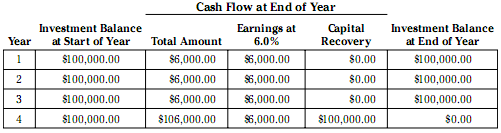On the above figure, turn your attention to the three columns under Cash Flow at End of Year. The first column is the total cash flow for the period (one year, in this scenario); the second column is for the earnings on the investment for the period (based on the ROI for the investment); and the third column is for capital recovery for the period. Capital recovery equals the excess of the total cash flow over the amount earnings for the period. In the first three years, capital recovery is zero because the cash flows equal earnings for the year. But in the fourth and final year, the investment generates \$106,000 total cash flow; the first \$6,000 of this is the amount of earnings for the year, and the remainder is capital recovery. The \$100,000 capital recovery in the final year exhausts the investment project; the investment venture is completed at this point. The entry cost of the investment has been fully recovered, and there are no more future cash flows.

### Scenario 2: ROI with Substantial Cash Flows Each Year

You invest \$100,000 today. The year-end cash flows from the investment are as follows:

year 1 = \$31,000; year 2 = \$29,500; year 3 = \$28,000; and year 4 = \$26,500.

What is the annual rate of return on investment for this scenario?

The annual rate of return on investment is 6 percent. Check out the following schedule, which uses the same template used on the scenario 1. There’s \$25,000 capital recovery every year, so the investment balance decreases year-to-year. This decrease may or may not be attractive to you as an investor because you recover your capital quicker, but you earn less on the investment year-to-year.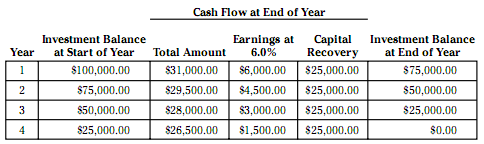Earnings are taken out of cash flow for the period first, before capital recovery is determined. In other words, the amount of capital recovery each year is subordinate to earnings. Earnings come first, and capital recovery is second.

### Scenario 3: ROI with Zero Cash Flow Until Final Year

You invest \$100,000 today. The year-end cash flows from the investment are as follows:

year 1 = \$0; year 2 = \$0; year 3 = \$0; and year 4 = \$136,048.90.

What is the annual rate of return on investment for this scenario?

The annual rate of return on investment is 8 percent. Check out the following schedule. Even though no cash flow is received in the first three years, earnings are assigned to each of the first three years at the rate of 8 percent per year. The negative numbers for the first three years in the capital recovery column mean that the imputed earnings are, in effect, compounded or added into the investment balance. For instance, at the start of year 2, the investment balance includes the amount of non-received earnings for the first year.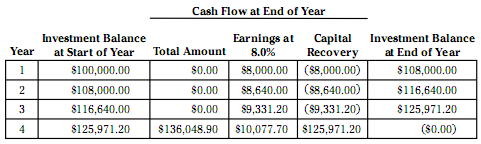This scenario brings out an exceedingly important point: There’s no cash flow until the end of the investment, so from a strict cash flow point of view, you can argue  that the annual earnings for the first three years are zero. Then in the fourth year, the investment earns 36.05 percent ROI (\$36,048.90 cash flow in excess of the entry cost / \$100,000.00 entry cost = 36.05 percent).

In summary, you can make the case that the annual ROI is as follows: year 1 = 0.0 percent; year 2 = 0.0 percent; year 3 = 0.0 percent; and, year 4 = 36.05 percent.

Well . . . you could argue this point of view, but you’d be lonely because no one does it this way.

In the world of finance, the ROI for scenario 3 is measured at 8 percent per year. The standard method for determining the ROI on the investment assumes that the annual earnings are theoretically received in cash but then immediately reinvested. Thus, you see the compounding effect from year to year; the investment balance increases year-to-year by the amount of reinvested earnings.

Don’t think that because the investment earns 8 percent ROI each year, you actually have any cash flow from the investment. You don’t!. In short, the 8 percent ROI solution is a convenient way to express the annual rate of growth in the value of the investment. It’s rather arbitrary, but it’s the way things are done.

### Scenario 4: ROI with Irregular Cash Flows [Both Positive And Negative]

You invest \$100,000 today. The year-end cash flows from the investment are as follows:

year 1 = negative \$15,000; year 2 = negative \$25,000; year 3 = \$50,000; and year 4 = \$141.625.

What is the annual rate of return on investment for this scenario?

The annual rate of return on investment is 10 percent. Check out the following schedule, which uses the same template. This sort of investment may not be for you because you put \$100,000 in the investment to get it started, and then at the end of the first and second years, you put additional money in the investment. These additional payments into the investment are called “negative cash flows”.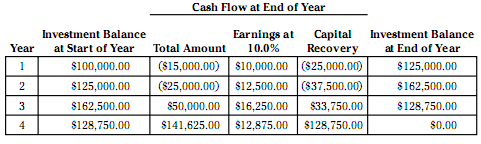Would you make this investment?

You would have to pump \$140,000 into the project before you see any positive cash flow at the end of year 3. You may or may not be in a position to do this. On the other hand, the investment yields 10 percent annual ROI, which is pretty good. Generally, most investments involving negative cash flows are riskier and, therefore, demand a higher than average ROI to justify taking on the risks.

### Scenario 5: ROI with Market Value–Driven Investments

You invest \$100,000 today in a mutual fund. All dividends are reinvested in additional shares. The performance of your investment over four years is as follows: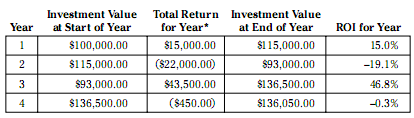Note: * Includes cash income and change in market value of investment portfolio.

The Total Return for Year column includes cash income and change in the market value of the investment portfolio.

How would most mutual funds advertise ROI performance for the four years in this scenario?

Instead of presenting a year-by-year ROI summary, which would show wild swings, a mutual fund with the investment performance shown in the scenario would advertise that it earned an average annual 8 percent ROI over the last four years.

The mutual fund argues that the performance of the fund is equivalent to having invested \$100,000 and earning a level 8 percent annual ROI. Indeed, earning 8 percent per year causes the investment to increase from \$100,000.00 to \$136,050.00 four years later, which is the ending value of the investment (see the preceding schedule of returns). In other words, the mutual fund says that if the investment had grown in value at a steady rate of 8 percent per year, then the end result would be the same. This claim is true, as the following schedule shows, but it certainly isn’t the whole story. Investors need to be informed about the wide swings in ROI year to year: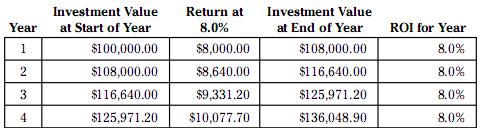Are you looking for easy accounting tutorial? Established since 2007, Accounting-Financial-Tax.com hosts more than 1300 articles (still growing), and has helped millions accounting student, teacher, junior accountants and small business owners, worldwide.